Next: Conclusions to Chapter 4 Up: Criticism of the conventional interpretation Previous: The Compton effect   Contents

The above described possibility of nonplanar motion even for two real bodies must be taken into account in the problem of the displacement of Mercury's perihelion (nobody made this).

Let us make some supplementary remark. In deriving the relativistic expression for the momentum "it is proved" that the momentum should be directed along the velocity, otherwise it would be indefinite. However, this reasoning is not rigorous in any way with respect to a single particle, because in a system, where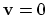, the direction of momentum is indefinite too. The classical expression for momentum follows from the Euclidean nature (homogeneity, isotropy) of space and from the invariance of mass. Following the minimum necessity principle, one can keep the classical expression both for the direction, and for the magnitude of particle's momentum. Then all relativistic changes will be revealed in changing the expression for energy. Simply, it is necessary to remember that for charged particles the field can also possess nonzero energy and momentum. And only the collision of neutral particles without internal degrees of freedom can be strictly elastic.

One more supplementary remark. In the book  (exercise 65 - "the momentum without mass") the platform on caster wheels is considered. At one of its ends the motor with accumulator is installed, which rotates, by means of a belt-driven transmission (through the whole platform), the caster wheel with vanes in water at the other end of a platform. As a result, the electrical energy of the accumulator transfers from one end of a platform into the thermal energy of water at the other end of a platform. Again, now we deal with the loss if determinacy (with non-objectivity): for "saving" SRT various observers should draw various artificial conclusions about the paths and rates of energy (mass) transfer. For example, according to SRT, the observer on a platform should assign the energy (mass) transfer to the belt-driven transmission. And if we shall leave to him open for observation only two small chunks of a belt, then in what and how this mass transfer can be confirmed experimentally? The classical physics attitude is more legible: if one body influences the second one, then the committed work is determined by the product of acting force on the relative path:or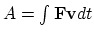, where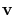is the relative velocity. For example, under an effect of the friction force a moving body stops. The kinetic energy of a body relative to the surface will be numerically equal to the work of the friction force and is numerically equal to the amount of released heat. These quantities are invariant (do not depend on the observation system).

Now we shall make a methodical remark on confirmation of relativistic formulas. The accuracy of experiments in microscopic world's physics is low, as a rule, in a separate measurement act. However, this accuracy is artificially increased by choosing the events "needed for the theory" and by subsequent statistical processing the results (adjustment under the theory). Unlike the classical field of investigation, nobody measures directly the value of velocity of particles in relativistic ranges of velocities (as well as the mass cannot be directly measured, but it is only possible for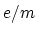with using definite theoretical interpretations and appropriate calibration of instruments). Therefore, it is impossible to substitute, in the explicit form, quantities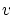and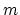into calculated (!) values of energy and momentum and verify the conservation laws of SRT. Even if one determines experimentally some nearly-kept numerical quantities, the literal expression for energy and momentum can be extracted from these values by many various techniques with different results. And, you see, even the numerical values of energy and momentum have been measured indirectly (again, we are dealing with theoretical interpretations).

If some object possesses a speed which is large than the speed with which you can moves your hand, then you cannot accelerate this object with the hand; however, the speed of a collision during a contrary motion will be defined by the sum of the velocities. The situation will be quite analogous if we, using the electromagnetic field, will try to accelerate particles flying nearly the speed of transmission of electromagnetic interactions (the efficiency of acceleration will be small); but again the velocities of particles will be added for the head-on collision in an additive manner. Consider the following mental experiment. Let three observers at points A, B and C be placed at one stright line. Let the distance |AB| be equal to the distance |BC|. A periodical synchronizing source O is placed at the middle perpendicular OB. The distance R=|OB| is very great. Note that all points are in the relative rest and this synchronizing procedure (from the remote source) is valid in the SRT also. As the result of such the synchronization, a precision of the synchronization at the all three points A, B, C can be made an arbitrary small value by choosing the appropriate large value of R. Let there be radioactive sources at end points A and C radiating particles at speed 0.9c. With receiving the first synchronizing signal from O, screens at points A and C are simultaneously opened. Particles from the points A and C fly to the central point B towards each other. The observer at the point B will see that the space between the two beams of particles are to be "eating up" with the speed of 0.9c+0.9c=1.8c. With the same speed particles will "get one's teeth into other's body" (to prove the validity of calculations, an instant of the collision can be arrived just in time of the second synchronizing signal). Just this speed is the speed of the particle's collision. But the relativistic law of the velocity addition bears no relation to the reality at all. In the relativity theory many additional, but really fictive, reaction channel arise as the result of erroneous ascribing reactions at different conditions to the reactions at the same conditions (parameters of the collision).

There arises a question: can superluminal velocities (for usual particles, but not for fairy-tale "tachions") be obtained and be observed by the real resting observer? We answer in such a manner: it is almost improbably that particle's speeds would be principally limited to the light speed (in line with the above mentioned, more precisely - even to the double speed of light). It could be observed under several conditions only: first, true elementary particles must be absent in the Nature; second, all the World must possesses the exclusively electromagnetic nature and must strictly obey to the Maxwell equations. However, there is good reason to believe that 1) true elementary particles exist, that 2) in addition to electromagnetic interactions, there exist the other interactions (at least three additional ones) in the Nature, and that 3) even the electromagnetic interactions themselves cannot be exclusively described by the modern form of the Maxwell equations (this fact was pointed out even by Ritz; remember also the fact itself of the birth of the quantum mechanics). In practice, it can be proposed the following. Consider collisions on rarefied contrary beams of particles flying practically with the speed of light. At strictly head-on collisions of true elementary particles of the same charges but of rather different masses (protons and positrons, for example), the more light particles will possess speeds between the double and triple speed of light (depending on speeds of the non-point particles at the moment of their direct contact) at scattering on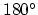. Of course, the probability of such the events is small (but not zero!), since small deviations from the strictly head-on collision lead to essential change in speeds of particles. It is more difficult to realize a repeated iteration of such the procedure (it is some analog of the Fermi acceleration) for obtaining large speeds, but it is possible in the Universe.

In studying collisions with particles being "at rest" the question arises: where so many resting particles have been found from? And how had this fact been verified (since this circumstance can relate to determination of collision and scattering angles, of an aiming parameter, etc.)?

We concentrate our attention on the fact that the energy acquired in a unit time by a particle in its passage through an electromagnetic field region can be described by one and the same formulaboth in the classical and in the relativistic cases . This fact provide one of causes for the "near-successful" calculation of accelerators. The same "events" and readings of devices are simply related to different scales of energy (more precisely, to different combinations of letter symbols) in the classical and relativistic cases.

SRT bears no priority relation to explaining the presence of momentum in a photon. Any particle (including a photon) is detected from its interaction with other particles, that is, actually, from the momentum transfer. In the modern interpretations, the experimental basis for defining the presence of photon's momentum are Lebedev's experiments on measuring the pressure of light. The literal expression for a kinetic energy of photon can be elementary deduced from the general definition: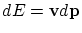(from the general equations of motion). If we take into account that the photon moves at a speed of light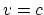, then after integration we obtainwithout any SRT's ideas. However, this formula can be applicable to light in vacuum only (but not in other mediums).

Also fully unsatisfactory is the semi-classical derivation of the Einstein formula :. First, the notion of the center of masses is contradictory in SRT. Second, for some reason one remembers about acoustic waves in SRT only when they are unessential (distract from apparent paradoxes). But these waves bear some relation to the given situation. Let at the ends of a homogeneous tube of lengthand mass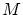(Fig. 4.11)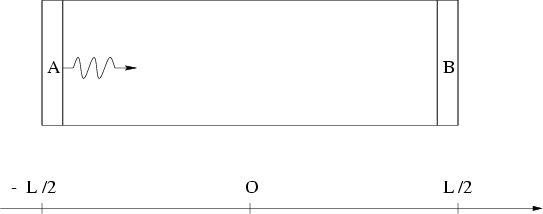there are bodies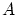andof negligible mass  (we take, for example, the monomolecular layers of the same substance). Let the atoms of layerbe at exited state. The following "circular process" is considered in . At first, bodyemits a short light pulse in the direction of body. It is stated that the tube will begin to move as a whole. But this is not the case. Let the lengthcm. The emitted pulse will make bodyto bend and move to the distance of about intermolecular one from tube's molecules retaining it. Then the elastic force will arise, which tends to return the lost equilibrium. As a result, the complicated system of longitudinal and transversal oscillations will propagate along a tube. During the time, for which the light will reach body, these acoustic waves will pass the distance not greater than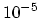cm (since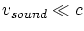). A similar process will be repeated with body. Thus, the oscillating tube will extend from center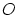in opposite directions (to the side of body- to a slightly greater distance), until the acoustic waves will cancel each other and the equilibrium will establish. But even this complicated real process does not matter, indeed. Further on  bodywith absorbed energy is brought into contact with bodyby internal forces; bodyreturns energy back to bodyand returns to its place (and then the mathematical symbols are written). One moment! Third, in what manner could bodytransfer electromagnetic excitation energy without transferring momentum? Besides, it could be only the light pulse (otherwise according to the second thermodynamic law not all energy could transfer to body). But in such a case we would simply have a mutually reciprocal momentum transfer by means of light, and no global conclusions follow from this situation. The given problem is similar to the classical problem on throwing a ball in a boat from one person to another. The ball has a mass, and in flight it also possesses nonzero momentum and energy. The value of mass enters expressions for a momentum and kinetic energy, but no "all-universe" conclusions follow from this situation. The thing sought in  can be obtained much easier. From the general expressionwe have for light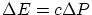. If we introduce, in a classical manner, for a photon the mass of motion, then fromfollows the only possibility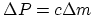. As a result, without any mental SRT ideas, we have. However, fourth, this result (irrespective of the method of its derivation) relates to the electromagnetic energy only and not to anything more (at least, there are no proofs of generality of the result).

An incorrect procedure may occur to be also the searching for solutions in SRT to an accuracy of up to some expansion in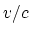. The rejected terms can cardinally change the form of a solution. The field of applicability of the approximate solution in time can occur to be such small, that the approximate solution will not have any theoretical and practical value (but how can it be detected without knowing the behavior of a true function?). Also doubtful is to derive the averaged solution from approximate one. A trivial example: it would seem formally, that in the Lorentz force it is possible to neglect the magnetic force containing. This is not the case, however: in the classical limit, instead of a real average drift of a particle at constant velocity perpendicular to both fields, we would obtain the accelerated motion along the field. In the relativistic limit  the velocities grows most rapidly also in the direction of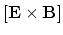. Apparently, due to this reason the approximate Lagrange functions, constructed in SRT up to some term in, can cause some problems, and the construction of an accurate Lagrange function is principally problematic in SRT. The limited nature of SRT results reveals itself in self-acceleration of charges under an effect of radiation reaction. The radiation is determined in the far-field zone and should not strongly depend on the processes occurring on particle scales: only re-evaluation of SRT rigorousness compels one to consider elementary particles as point-like ones.

Though the following methodical remark concerns kinematics first, it touches also upon the GRT and the relativistic dynamics as well. The problem is setted in : to describe the motion of the system under investigation, which is uniformly accelerated relative to the own inertial system (the latter is instantly in the rest relative the system being investigated). A reader can have the natural question: whether the motion, which is uniformly accelerated relative to one inertial system, can really be nonuniformly accelerated ralative to the other inertial systems or not? Unfortunately, in the SRT the situation turns out just the same (we are lucky that the relativity theory practically not use higher derivatives, except the description of radiation, otherwise we could see many new "peculiarities"). However, what will we have with the equivalence principle: in one inertial system there exists an equivalence to some gravitational field (constant), but in the other inertial system in the same space point were we be having the changed gravitational (physical!) field? To "see" the flight of cobble-stones as balloons, with what a speed must the observer fly? But if we will attach the dynamometer to such the uniformly accelerated rocket and hang up a weight to the spring, then, whether differently moving (but with some constant velocities) observers would see that the dynamometer pointer show different Arabic cipher or not?

We mention the well known paradox of a relativistic submarine (the SRT was stopped in choosing as "the Buridan's donkey" between two haystacks): from the viewpoint of an observer at the earth surface, the moving submarine must sink due to increasing it's density as a result of shortening of it's length; contrary, from the viewpoint of an observer at the submarine, the latter must surface due to increasing of density of surroundings water. It was needed to say some "magic pseudo-scientific spell", and relativists chose to allege either an acceleration process or a curved space in increased gravitational field, that is they sent off to the GRT again. Possibly, it can be written as an epitaph: "the SRT made efforts to fill immensity, but never possessed an own subject for investigation". We re-formulate the present paradox in different manner to evidently see that gravitation bears no relation to this case. Let the following ANSWER be known from the viewpoints of both observers. At the usual earth conditions (i.e. at the weak gravitational field!), an usual submarine had successfully passed a path between two ships with a constant (non-relativistic!) speed at a given fixed depth (in transparent water). Now the question will be: what must declare different moving relativistic observers from the SRT viewpoint? Since the SRT "worked" with the exchange of light signals only, then, naturally, all SRT "claims" must be seen by relativistic observers just with the help of the light by itself. When they will see "it"? Evidently, it will be in just the time, when the light emitted in the moment of an "event" will reach them (as relativists claim, there not exist instantaneous relations). Let from the distance of 20 billions light years two observers (in moving spaceships) will see in the direction of our submarine in 20 billions years (when, "possibly", our submarine and ships will no longer exist), and let the observers catch the signals from the remoute event. Let the observers will be moving with a speed close to the speed of light, the one observer -- in the direction of the submarine's course, and the second one -- in the opposite direction. It turns out that opinions of these two observers (the submarine was sunk or surfaced) must be different according to the SRT (as the result of a velocity addition). And these observers cannot believe even the our arrived spacecraft (with some delay to not disturb the relativistic sleep) with the report that "the submarine had successfully executed the order at the GIVEN DEPTH". We would like to believe relativists: it may be that Vasilii Ivanovich Chapaev did not drown, in the case if some "right alien", flying with a "right velocity" at some "right time", will look at that remote event.

Of course, all losses of objective characteristics of SRT (which are presented here only for completeness of the picture) look simply as "student's fittings" as compared to the logical gaps and contradictions existing in SRT. It is absolutely strange the stock phrase spreaded by relativists as if the SRT is simply a new geometry and, therefore, it is allegedly noncontradictory. Possibly, they simply do not sense even the subject of physical investigations by itself (the physics studies causes of phenomena and concrete mechanisms directly influencing on the phenomenon under investigation). Of course, to obtain mathematical solutions, different transformations of coordinates are frequently used in physics (conformal ones, for example). In particular, the Lorentz transformations (but with the speed of sound) can be used for solving some problems in the acoustics (just since they are an invariant). However, if somebody were claim that the real change of the all Universe from the outer region into the inner one of a circle follows from correctness of some solutions, all physicists would understand "the adequate place" for such the claim. But if other but Very Big Relativistic Scientist claims that the all Universe was compressed when He walked to the nearest bakery, then many "yes-mans" will confirm this rubbish (possibly, these poor devils were rather deprived from childhood - the tale "Bare King" was not read to them).

From author's standpoint the most consistent attitude is a principal recognition of the results of relativistic dynamics and electrodynamics as approximate ones, to an accuracy provided by the experiment. One should not overestimate the possibilities of purely theoretical techniques and to overload the physics with globalisms. It is namely this reason and insufficient substantiation of relativistic experiments, why the author does not try to offer any alternative theory. At present, the theory should analyze and generalize those experiments, which have been carried out particularly in the region of high velocities.Next: Conclusions to Chapter 4 Up: Criticism of the conventional interpretation Previous: The Compton effect   Contents
Sergey N. Arteha